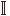# Maths - Examples of Cubical Types

Higher inductive types in cubical type theory.

### Circle and Spheres (Z)

FormationS1
(Z)
 S1:U

The circle has two constructors:

• a point
• a loop path
Introduction
 base:S1
()
 r:loop r :S1
()
Elimination
 x :S1P(x) b:P(base) l :Pathi P(loop i)b b u:S1
S1 -elimx.p b l u : P(u)
()

where:

Pathi is a dependant path type

 From CubicalTT ```data S1 = base | loop [ (i=0) -> base , (i=1) -> base]```

### Torus

 From CubicalTT ```data Torus = ptT | pathOneT [ (i=0) -> ptT, (i=1) -> ptT ] | pathTwoT [ (i=0) -> ptT, (i=1) -> ptT ] | squareT [ (i=0) -> pathOneT {Torus} @ j , (i=1) -> pathOneT {Torus} @ j , (j=0) -> pathTwoT {Torus} @ i , (j=1) -> pathTwoT {Torus} @ i ] -- Torus = S1 * S1 proof -- pathTwoT x -- ________________ -- | | -- pathOneT y | squareT x y | pathOneT y -- | | -- | | -- ________________ -- pathTwoT x -- pathOneT is (loop,refl) -- pathTwoT is (refl,loop)```

### n-sphere (Suspensions)

 From CubicalTT ```-- Suspension. Definition of the circle as the suspension of bool and -- a proof that this is equal to the standard HIT representation of -- the circle. data susp (A : U) = north | south | merid (a : A) [ (i=0) -> north , (i=1) -> south ] -- n-spheres sphere : nat -> U = split zero -> bool suc n -> susp (sphere n)```

### Propositional Truncation

 From CubicalTT ```data pTrunc (A : U) = inc (a : A) | inh (x y : pTrunc A) [(i=0) -> x, (i=1) -> y]```

### Pushouts

Where I can, I have put links to Amazon for books that are relevant to the subject, click on the appropriate country flag to get more details of the book or to buy it from them.Computation and Reasoning - This book is about type theory. Although it is very technical it is aimed at computer scientists, so it has more discussion than a book aimed at pure mathematicians. It is especially useful for the coverage of dependant types.